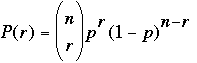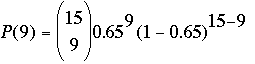SEARCH HOMEMath Central Quandaries & QueriesQuestion from Rana: According to one study, it has been claimed that 65% of all single men in Montreal would welcome a woman taking the initiative in asking for a date. You decide to challenge this study by performing a little experiment of your own. You randomly select 15 single men from around Montreal and ask them if they would be comfortable with a woman asking them out for a date. If the claim made by the study was true, what is the probability that: a) Exactly 9 men would say yes? b) Exactly 6 men would say no? c) More than 11 men would say yes? d) At most 4 men would say no?Hi Rana,

I can get you started by showing you how to solve part a).

If the probability that a single male would welcome a woman taking the initiative in asking for a date is 0.65 and you randomly and independently select 15 single men and ask them if they would welcome a
woman taking the initiative in asking for a date then you are working with a binomial distribution. The sample size is n = 15 and the probability of success (a man answering yes to your question) is 0.65. The binomial distribution then says that the probability of r successes isFor part a) you have r = 9 and hence the probability that exactly 9 men say yes isPennyMath Central is supported by the University of Regina and The Pacific Institute for the Mathematical Sciences.